## Rufus collected 18 pounds of aluminum cans to recycle. He plans to collect an additional 2 pounds each week. He wants to collect a total of

Question

Rufus collected 18 pounds of aluminum cans to recycle. He plans to collect an additional 2 pounds each week. He wants to collect a total of 42 pounds of aluminum cans. Let x represent the number of weeks Rufus collects aluminum cans.

in progress 0
6 months 2021-08-26T09:57:06+00:00 1 Answers 31 views 0

## Answers ( )

12 weeks

Step-by-step explanation:

Given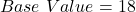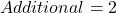weekly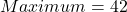Required

Determine the number of weeks

Represent the maximum number of pounds with y and the number of weeks with x.

Such that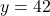From the given parameters, the relationship between y and x is as follows: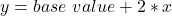Substitute 42 for y and 18 for base value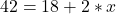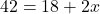Subtract 18 from both sides.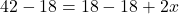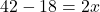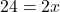Divide both sides by 2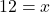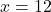Hence, the number of weeks is 12